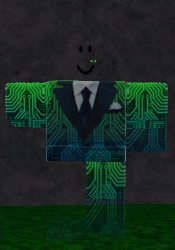# The NPC character doesn't fully disappear

Hello everyone.

I was making a trigger that will hide an NPC character however I ran into an issue:as you can see the character is not fully hidden (ignore the face).
here is the script:

``````local Part = script.Parent
local Model = Part.Parent
local debounce = false

Part.Touched:Connect(function(hit)
if debounce == false then
local humanoid = hit.Parent:FindFirstChild("Humanoid")
if humanoid then
local x = 0
debounce = true
for i,v in ipairs(Model:GetChildren()) do
if v:IsA("Part") or v:IsA("MeshPart") then
coroutine.wrap(function()
repeat
print(v)
print(4)
v.Transparency = v.Transparency + 0.1
wait(0.1)
x = x + 1
until x == 10
x = 0
end)(v)
end
end
end
end
end)
``````

I’m not quite familiar with coroutine yet so any help will be good2 Likes

simple its the clothes and the decal! just make em go manually or if u want automatically
here

``````local Part = script.Parent
local Model = Part.Parent
local debounce = false

Part.Touched:Connect(function(hit)
if debounce == false then
local humanoid = hit.Parent:FindFirstChild("Humanoid")
if humanoid then
local x = 0
debounce = true
for i,v in ipairs(Model:GetDescendants()) do
if v:IsA("Part") or v:IsA("MeshPart") or v:IsA("Decal") or v:IsA("Shirt") or v:IsA("Pants") then
coroutine.wrap(function()
repeat
print(v)
print(4)
v.Transparency = v.Transparency + 0.1
wait(0.1)
x = x + 1
until x == 10
x = 0
end)(v)
end
end
end
end
end)
``````
2 Likes

if u can see he is using a something like a tween effect destroying would make them go in a blink of an eye

1 Like
``````local Tweens = game:GetService("TweenService")

local Part = script.Parent
local Model = Part.Parent

local Debounce = false

local function MakeInvisibleRecursive(Object)
for _, Instance in ipairs(Object:GetChildren()) do
if Instance:IsA("BasePart") then
local Tween = Tweens:Create(Instance, TweenInfo.new(0.5, Enum.EasingStyle.Linear), {Transparency = 1})
Tween:Play()
local Face = Instance:FindFirstChild("face")
if not Face then continue end
MakeInvisibleRecursive(Face)
end
elseif Instance:IsA("Decal") then
local Tween = Tweens:Create(Instance, TweenInfo.new(0.5, Enum.EasingStyle.Linear), {Transparency = 1})
Tween:Play()
elseif Instance:IsA("Accessory") then
MakeInvisibleRecursive(Instance)
elseif Instance:IsA("Tool") then
MakeInvisibleRecursive(Instance)
end
end
end

Part.Touched:Connect(function(hitPart)
if Debounce then return end
local hitModel = hitPart:FindFirstAncestorOfClass("Model")
if not hitModel then return end
local hitHuman = hitPart:FindFirstChildOfClass("Humanoid")
if not hitHuman then return end
if hitHuman.Health <= 0 then return end
Debounce = true
MakeInvisibleRecursive(Model)